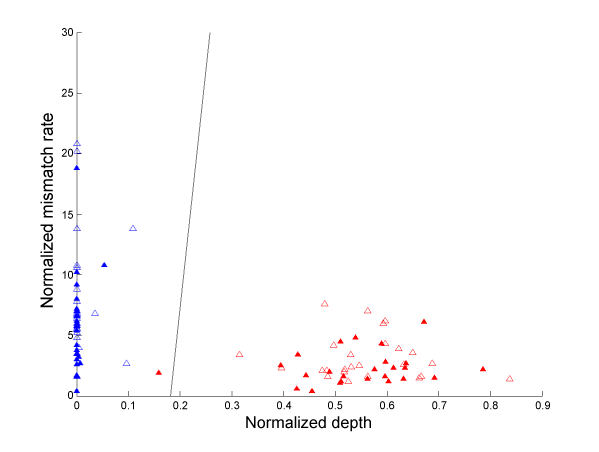Figure 5: Normalized depth versus mismatch rate for 50% and 100% indels. The empty triangles are the training data, the filled triangles are the test data. The X axis represents the normalized mismatch rate  m% and the Y axis represents the normalized sequencing depth d% . The red points are the 50% spiked indels, the blue points are the 100% spiked indels. One of the 50% spiked insertions is misclassified. The black line is the linear classification boundary as defined by the following: 197*  m% +0.5* d% + 77=0.